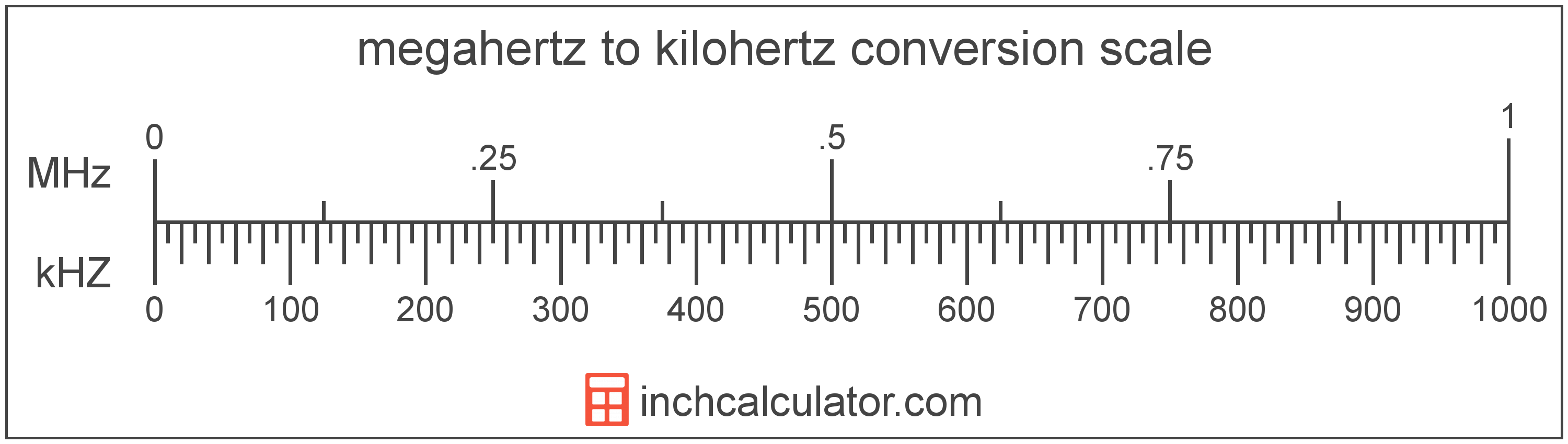Convert Kilohertz to Megahertz

Enter the frequency in kilohertz below to get the value converted to megahertz.

Results in Megahertz:1 kHZ = 0.001 MHz

How to Convert Kilohertz to MegahertzTo convert a kilohertz measurement to a megahertz measurement, multiply the frequency by the conversion ratio. One kilohertz is equal to 0.001 megahertz, so use this simple formula to convert:

megahertz = kilohertz × 0.001

The frequency in megahertz is equal to the kilohertz multiplied by 0.001.

For example, here's how to convert 5 kilohertz to megahertz using the formula above.
5 kHZ = (5 × 0.001) = 0.005 MHz

Kilohertz and megahertz are both units used to measure frequency. Keep reading to learn more about each unit of measure.

Kilohertz

Kilohertz is a measure of frequency equal to one thousand cycles per second.

The kilohertz is a multiple of the hertz, which is the SI derived unit for frequency. In the metric system, "kilo" is the prefix for 103. Kilohertz can be abbreviated as kHZ, for example 1 kilohertz can be written as 1 kHZ.

Megahertz

Megahertz is a measure of frequency equal to one million cycles per second.

The megahertz is a multiple of the hertz, which is the SI derived unit for frequency. In the metric system, "mega" is the prefix for 109. Megahertz can be abbreviated as MHz, for example 1 megahertz can be written as 1 MHz.

Kilohertz Measurements and Equivalent Megahertz Conversions

Common kilohertz values converted to the equivalent megahertz value
Kilohertz Megahertz
1 kHZ 0.001 MHz
2 kHZ 0.002 MHz
3 kHZ 0.003 MHz
4 kHZ 0.004 MHz
5 kHZ 0.005 MHz
6 kHZ 0.006 MHz
7 kHZ 0.007 MHz
8 kHZ 0.008 MHz
9 kHZ 0.009 MHz
10 kHZ 0.01 MHz
11 kHZ 0.011 MHz
12 kHZ 0.012 MHz
13 kHZ 0.013 MHz
14 kHZ 0.014 MHz
15 kHZ 0.015 MHz
16 kHZ 0.016 MHz
17 kHZ 0.017 MHz
18 kHZ 0.018 MHz
19 kHZ 0.019 MHz
20 kHZ 0.02 MHz
21 kHZ 0.021 MHz
22 kHZ 0.022 MHz
23 kHZ 0.023 MHz
24 kHZ 0.024 MHz
25 kHZ 0.025 MHz
26 kHZ 0.026 MHz
27 kHZ 0.027 MHz
28 kHZ 0.028 MHz
29 kHZ 0.029 MHz
30 kHZ 0.03 MHz
31 kHZ 0.031 MHz
32 kHZ 0.032 MHz
33 kHZ 0.033 MHz
34 kHZ 0.034 MHz
35 kHZ 0.035 MHz
36 kHZ 0.036 MHz
37 kHZ 0.037 MHz
38 kHZ 0.038 MHz
39 kHZ 0.039 MHz
40 kHZ 0.04 MHz

More Kilohertz Frequency Conversions

Frequency
Convert to Hertz
1 kHZ is equal to 1,000 hertz
Convert to Gigahertz
1 kHZ is equal to 1.0E-6 gigahertz
Convert to Terahertz
1 kHZ is equal to 1.0E-9 terahertz
Angular Frequency
Convert to Cycles Per Second
1 kHZ is equal to 1,000 cycles per second
Convert to Revolutions Per Minute
1 kHZ is equal to 60,000 revolutions per minute
Convert to Radians Per Second
1 kHZ is equal to 6,283.19 radians per second
Convert to Degrees Per Second
1 kHZ is equal to 360,000 degrees per second